1.matplotlib简介

2.图形组成元素的函数用法

2.1. figure()：背景颜色

2.3 xlabel()和 ylabel()：设置 x，y 轴的标签文本

2.4 grid()：绘制刻度线的网格线

2.5 axhline()：绘制平行于 x 轴额度水平参考线

2.6 axvspan()：绘制垂直于 x 轴的参考区域

2.7 xticks(),yticks()

2.8 annotate()：添加图形内容细节的指向型注释文本

2.9 bbox：给标题增加外框

2.10 . text()：添加图形内容细节的无指向型注释文本(水印）

2.11. title()：添加图形内容的标题

2.12. legend()：标示不同图形的文本标签图例

2.13 table()：向子图中添加表格

3. 完整代码显示

4.折线图的线条风格

5. 常用颜色缩写

6.总结

## 1.matplotlib简介

matplotlib 库是 Python 中绘制二维和三维图表的数据可视化工具

使用简单绘图语句实现复杂绘图效果
以交互式操作实现渐趋精细的图形效果
使用嵌入式 LaTex 输出具有印刷级别的图表、科学表达式和符号文本
对图表的组成元素实现精细化控制

pyplot：面向当前图

axes：面向对象

Pylab：沿用 matlab 风格

``````import matplotlib.pyplot as plt
import numpy as np``````

## 2.图形组成元素的函数用法

### plot()：展示变量的趋势变化

使用方法：plt.plot(x, y, c，ls, lw, label, alpha, **kwargs)
x，y：x，y 轴上的数值
c：设置颜色
ls：折线图的线条风格
lw：折线图的线条宽度
label：标记图形内容的标签文本
alpha：透明度
**kwargs：指定使用的是 line2D 属性

### 2.1. figure()：背景颜色

使 用 方 法 ： figure(num=None, figsize=None, dpi=None, facecolor=None,
edgecolor=None, frameon=True, FigureClass=Figure, clear=False, **kwargs)
num ：
如果此参数没有提供，则一个新的 figure 对象将被创建，同时增加 figure 的计数数值，此数值被保存在 figure 对象的一个数字属性当中。如果有此参数，且存在对应 id 的 figure 对象，则激活对于 id 的 figure 对象。如果对应 id 的 figur 对象不存在，则创建它并返回它。如果 num 的值是字符串，则将窗口标题设置为此字符串
figsize：以英寸为单位的宽高，缺省值为 rc figure.figsize (1 英寸等于 2.54 厘米)
dpi：图形分辨率，缺省值为 rc figure.dpi
facecolor：背景色
``````plt.figure(figsize=(10, 10))
x = np.linspace(0.05, 10, 1000)  # 在0.05到10的区间中，等差选取1000个，端点不属于
y = np.sin(x)
plt.rcParams['font.sans-serif'] = ['SimHei']
plt.rcParams['axes.unicode_minus'] = False
plt.plot(x, y,
color='red',
ls='-',
label='sinx')
plt.show()``````

###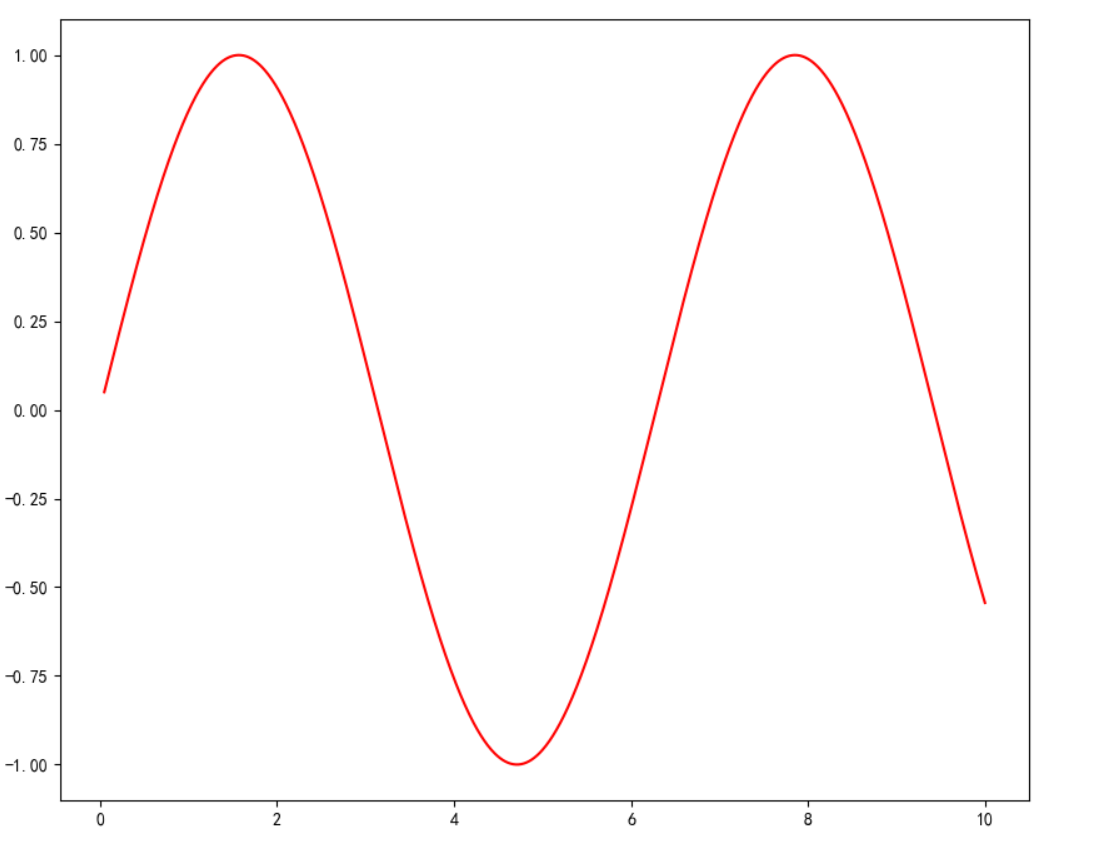2.2 xlim()和 ylim()：设置 x，y 轴的数值显示范围

使用方法：plt.xlim(xmin,xmax)
xmin：x 轴上的最小值
xmax：x 轴上的最大值

### 2.3 xlabel()和 ylabel()：设置 x，y 轴的标签文本

fontsize：数字或者（small，large，medium）
verticalalignment：距离坐标轴的位置（top，bottom，center，baseline）
hoizontalalignment：位置（center，right，left）
ratation：位置（vertical，horizontal，vertical）
bbox：添加边框

### 2.5 axhline()：绘制平行于 x 轴额度水平参考线

y：水平参考线的出发点
``````plt.figure(figsize=(10, 10))
x = np.linspace(0.05, 10, 1000)  # 在0.05到10的区间中，等差选取1000个，端点不属于
y = np.sin(x)
plt.rcParams['font.sans-serif'] = ['SimHei']
plt.rcParams['axes.unicode_minus'] = False
plt.plot(x, y,
color='red',
ls='-',
label='sinx')
plt.xlim(1, 10)
plt.ylim(-1, 1)
plt.xlabel('x轴')
plt.ylabel('y轴')
plt.grid(ls=':',
color='blue')  # 设置网格，颜色为蓝色
plt.axhline(0.5, color='green', lw=2, label="分割线")  # 绘制平行于x轴的水平参考线，绿色，名称
plt.show()``````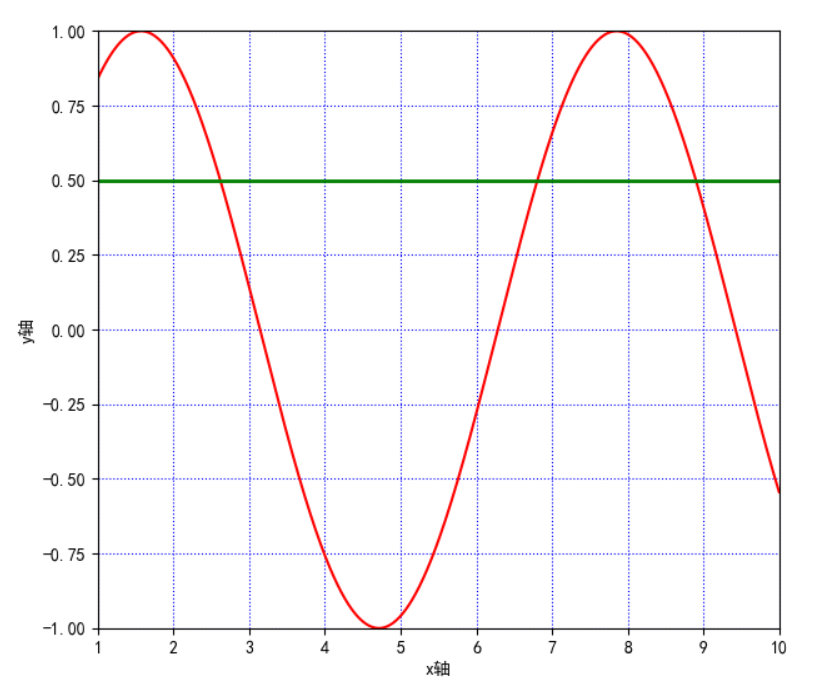（上图中绿色的线即为axjline（）添加的参考线）

### 2.6 axvspan()：绘制垂直于 x 轴的参考区域

xmin：参考区域的起始位置
xmax：参考区域的终止位置
facecolor：参考区域的填充颜色
alpha：参考区域填充颜色的透明度,[0~1]

``````plt.axvspan(xmin=2,
xmax=5,
facecolor='r',
alpha=0.2)  # 绘制垂直于x轴的参考区域``````

### 2.7 xticks(),yticks()

获取或设置当前 x 轴或 y 轴刻度位置和标签（即设置 x 或 y 轴的标 签）

``plt.xticks(list(range(0, 12, 1)))  # 调整刻度范围和刻度标签``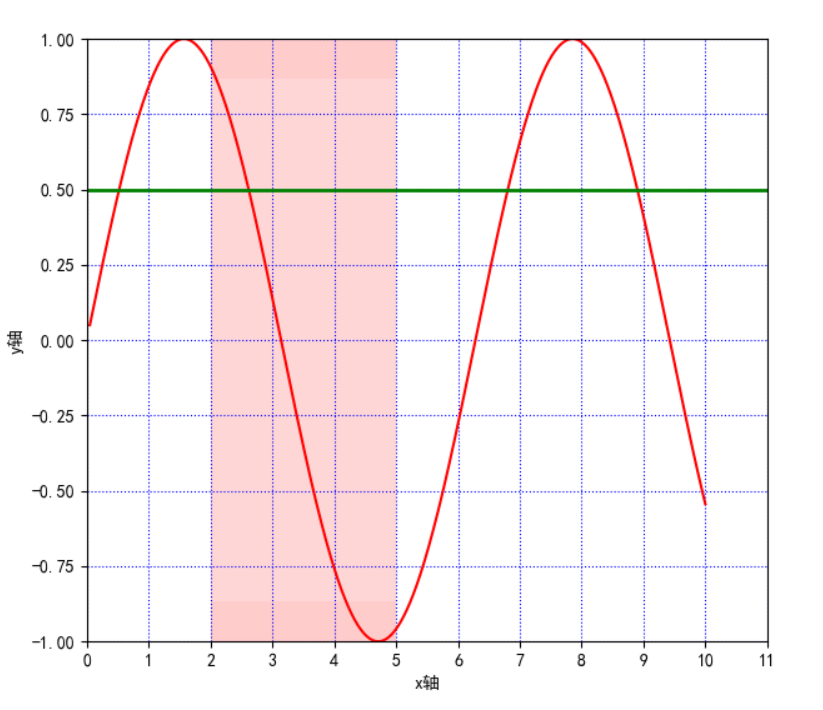### 2.8 annotate()：添加图形内容细节的指向型注释文本

s：注释文本内容

xy：被注释的坐标点

xytext：注释文字的坐标位置
weight：设置字体线形(Ultralight，light，normal，regular，book，medium，roman，semibold，demibold，demi,bold，heavy，extrabold，black）
color：设置字体颜色；也可以设置 RGB 或 RGBA 类型的颜色；但必须为[0,1]之间的浮点 数
xycoords= 参数如下
figure points：图左下角的点
figure pixels：图左下角的像素
figure fraction：图的左下部分
axes points：坐标轴左下的点
axes pixels：坐标轴左下的像素
data：使用被注释对象的坐标系统
arrowprops：箭头参数，参数类型为字典 dict
width：箭头的宽度
shrink：总长度的一部分，从两端“收缩”
facecolor：箭头颜色（如果设置了 arrowstyle 关键字，上面的参数都不可以用，可

-
->
-[
|-|
-|>
<->
<|-
<|-|>
fancy
simple
wedge
``````plt.annotate('local',
xy=(2, 1),
xytext=(0.5, 0.5),
weight='bold',
color='red',
xycoords="data",
arrowprops=
dict(arrowstyle="->", connectionstyle='arc3', color='b'),
bbox=
dict(boxstyle="rarrow",
fc="yellow",
ec='k',
lw=1,
alpha=0.5)
)``````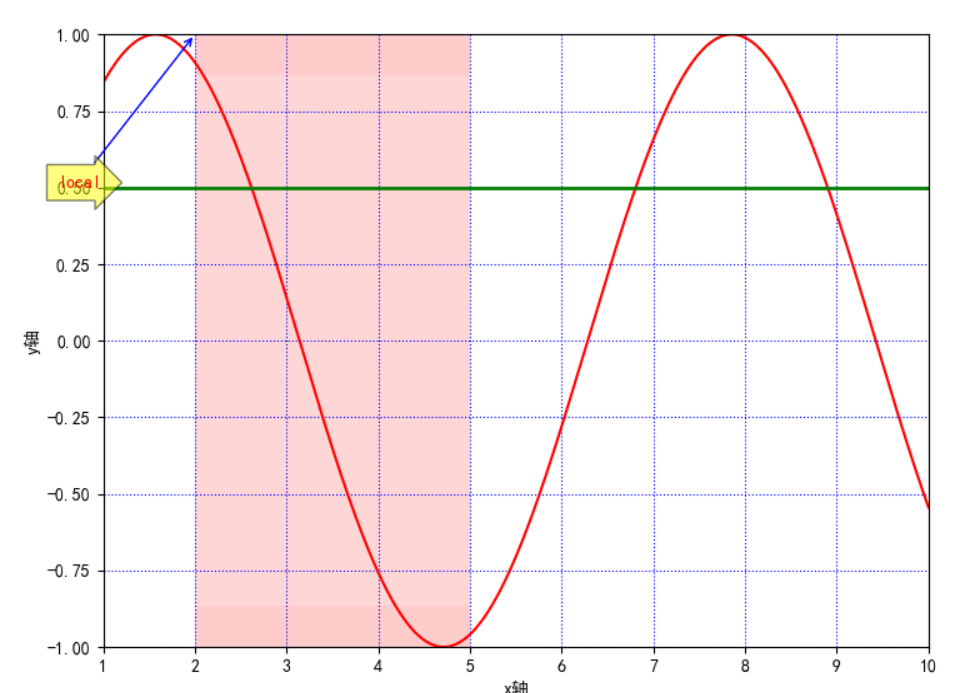这里的黄色箭头和蓝色细长线即为参数方法添加的参数，实际使用过程中根据自己的实际所需使用，可以认为添加对图像的一些解释

### 2.9 bbox：给标题增加外框

（boxstyle：方框外形；circle：椭圆；darrow：双向箭头；larrow：箭头向左；rarrow：箭

### 2.10 . text()：添加图形内容细节的无指向型注释文本(水印）

x,y：表示坐标轴上的值
weight：
ultralightlight
normal
regular
book
medium
roman
semibold
demibold
demi
bold
heavy
extrabold
black
xycoodrds：
figure points：图左下角的点
figure pixels：图左下角的像素
figure fraction：图的左下部分
axes points：坐标轴左下的点
axes pixels：坐标轴左下的像素

data：使用被注释对象的坐标系统

bbox：给标题增加外框
boxstyle：方框外形
circle：椭圆
darrow：双向箭头
larrow：箭头向左
rarrow：箭头向右
round：圆角矩形
round4：椭长方形
roundtooth：波浪形边框 1
sawtooth：波浪形边框 2
square：长方形
``````plt.text(1, 1,
"y=sinx",
weight='bold',
color ='b')``````

这里设置在坐标（1，1）上，即文字下面y=sinx的蓝色字段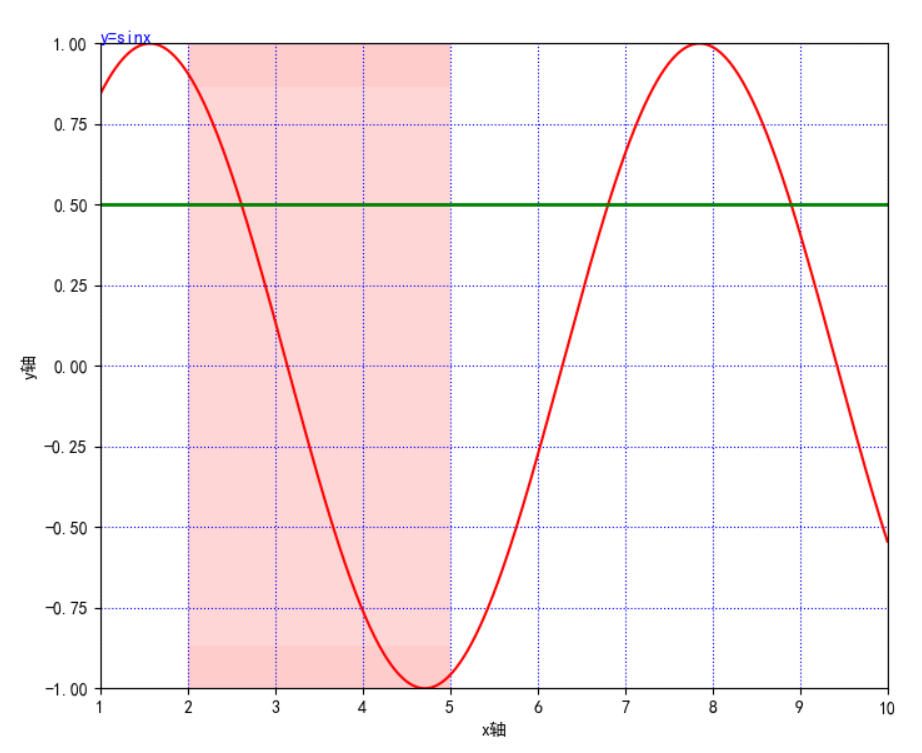### 2.11. title()：添加图形内容的标题

``plt.title("正弦函数")``

### 2.12. legend()：标示不同图形的文本标签图例

best
upper right
upper left
lower left
lower right
right
center left
center right
lower center
upper center
center
``plt.legend(loc="lower left")  # 设置图例位置``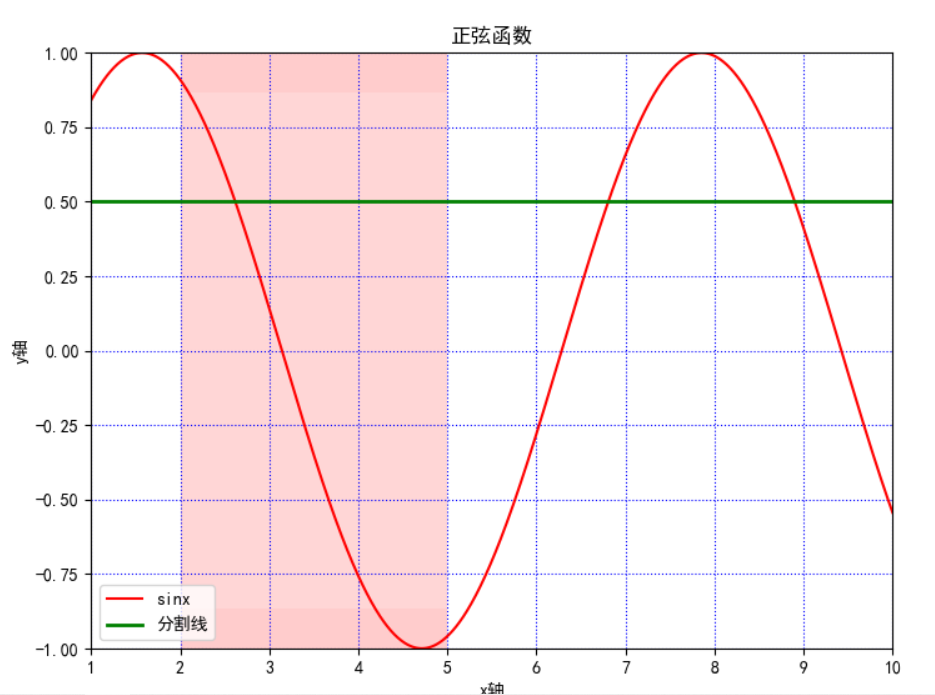### 2.13 table()：向子图中添加表格

plt.table(cellText=None, cellColours=None, cellloc='right' ,colWidths=None,
rowLabels=None, rowColours=None, collLabels=None, colColours=None,
collloc='center', loc='bpttpm', bbox=None, edges='closed', **kwargs)
cellText：表格单元格文本。类型为二维字符串列表
cellColours：表格单元格背景色。类型为二位颜色值列表
cellloc：表格单元格文本的对齐方式。默认值为right
left
right
center
colWidths：表格单元格宽度。类型为浮点数列表
rowLabels：表格行表头文本。类型为字符串列表
rowColours：表格行表头背景色。类型为颜色列表
colLabels：表格列表头文本。类型为字符串列表
colLoc：表格行表头文本对齐方式。默认 right
left
right
center
colColours：表格列表头背景色。类型为颜色列表
loc：单元格相对于子图的位置
bbox：绘制表格的边界框，如果此参数不为 None，将会覆盖 loc 参数
edges：单元格边线，该属性会影响各类单元格背景色
BRTL
open
closed
horizontal
vertical

## 3. 完整代码显示

``````import matplotlib.pyplot as plt
import numpy as np

plt.figure(figsize=(10, 10))
x = np.linspace(0.05, 10, 1000)  # 在0.05到10的区间中，等差选取1000个，端点不属于
y = np.sin(x)
plt.rcParams['font.sans-serif'] = ['SimHei']
plt.rcParams['axes.unicode_minus'] = False
plt.plot(x, y,
color='red',
ls='-',
label='sinx')
plt.xlim(1, 10)
plt.ylim(-1, 1)
plt.xlabel('x轴')
plt.ylabel('y轴')
plt.grid(ls=':',
color='blue')  # 设置网格，颜色为蓝色
plt.axhline(0.5, color='green', lw=2, label="分割线")  # 绘制平行于x轴的水平参考线，绿色，名称
plt.axvspan(xmin=2,
xmax=5,
facecolor='r',
alpha=0.2)  # 绘制垂直于x轴的参考区域
plt.axhspan(ymin=(-3**0.5)/2,
ymax=(3**0.5)/2,
facecolor='w',
alpha=0.2)

plt.legend(loc="lower left")  # 设置图例位置
plt.annotate('local',
xy=(2, 1),
xytext=(0.5, 0.5),
weight='bold',
color='red',
xycoords="data",
arrowprops=
dict(arrowstyle="->", connectionstyle='arc3', color='b'),
bbox=
dict(boxstyle="rarrow",
fc="yellow",
ec='k',
lw=1,
alpha=0.5)
)
plt.xticks(list(range(0, 12, 1)))  # 调整刻度范围和刻度标签
plt.text(1, 1,
"y=sinx",
weight='bold',
color ='b')
plt.title("正弦函数")
plt.show()``````

``````plt.rcParams['font.sans-serif'] = ['SimHei']
plt.rcParams['axes.unicode_minus'] = False``````

## 4.折线图的线条风格

``````   -：实线样式
--：短横线样式
-.：点划线样式
：：虚线样式
.：点标记
O：圆标记
V：倒三角标记
^：正三角标记
<：左三角标记
>：右三角表示
1：下箭头标记13
2：上箭头标记
3：左箭头标记
4：右箭头标记
S：正方形标记
p：五边形标记
*：星形标记
H：六边形标记
+：加号标记
X：x 标记
D：菱形标记
|：竖直线标记
_：水平线标记``````

## 5. 常用颜色缩写

``````b 蓝色
g 绿色
r 红色
c 青色
m 品红色·
y 黄色
k 黑色
w 白色``````

### 6.总结

很多参数有时候用不上，但要知道有，存在即合理，不同参数的作用功能不同，不要任何图都加太多参数，一般有图例、标题，xy轴的范围即可。

无论使用哪个，建议先试试，实践是检验真理的唯一标准！！！

有写的不好的地方希望读者见谅，我也在一步步摸索，有疑问欢迎在评论区讨论